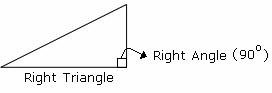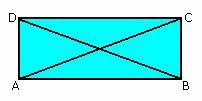## Definition Of Right Triangle

A triangle with one of its angles measuring 90° (i.e. a right angle) is called a Right Triangle.

Right triangle is also called as right-angled triangle.
In a right triangle, the side opposite to the right angle is the hypotenuse of the triangle and happens to be the longest side. The other two sides are called the
legs of the right triangle.
A right triangle always satisfies the Pythagorean theorem i.e. if h is the hypotenuse length, b is the base length, and p is the perpendicular length in a right triangle, then h2 = b2 + p2

### Examples of Right Triangle### Solved Example on Right Triangle

#### Ques: Which of the following figures is a right prism?A. 4
B. 2
C. 1
D. 3